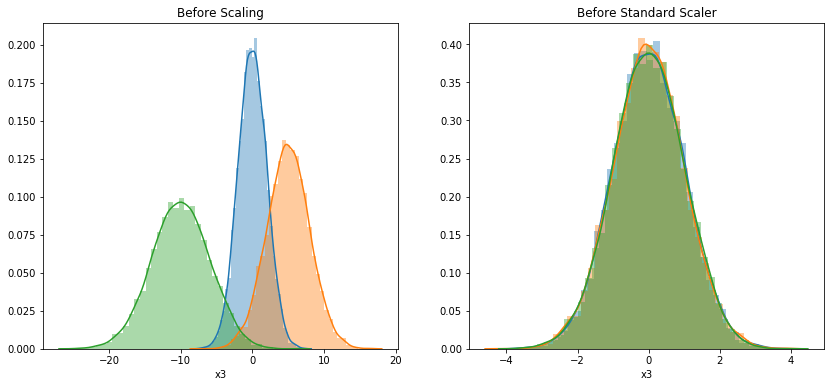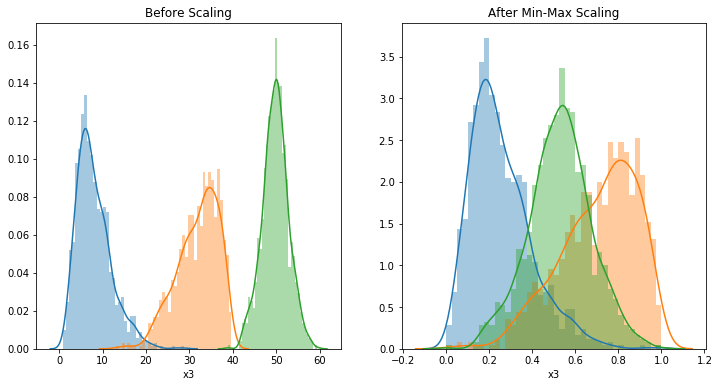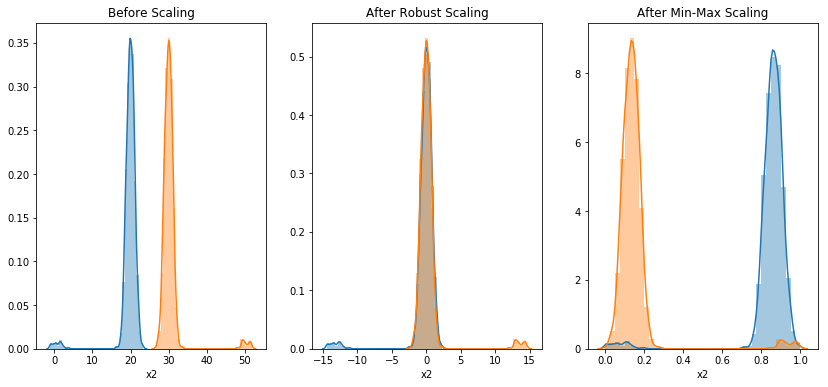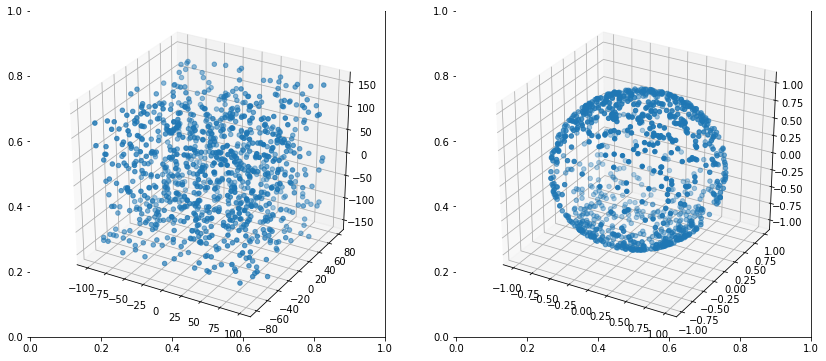# MLF - Scale, Standardize and Normalize Data

Last updated: April 28th, 2020# Scale, Standardize and Normalize data¶

Many machine learning algorithms work better when features are on a relatively similar scale and close to normally distributed. StandardScaler, MinMaxScaler, RobustScaler, and Normalizer are scikit-learn methods to preprocess data for machine learning.

The idea that there are different ways to represent predictors in a model, and that some of these representations are better than others, leads to the idea of feature engineering—the process of creating representations of data that increase the effectiveness of a model. In this lesson (and the followings) we make an introduction to feature engineering, which we will be back in details in feature engineering course.

Which method fits the best, if any, depends on your model type and your feature values. We will go through each of them and highlight the differences and similarities among these methods and help you learn when to reach for which tool.

In :
import numpy as np
import pandas as pd
import matplotlib.pyplot as plt
import seaborn as sns## Common scaler methods¶

In this lesson we will explore the sklearn.preprocessing package that provides several common utility functions and transformer classes to change raw feature vectors into a representation that is more suitable for the downstream estimators.

• StandardScaler
• MinMaxScaler
• RobustScaler
• Normalizer

To use any preprocessing techniques, we will first import the class, for example StandardScaler, and create a new instance.

Then, fit and transform the scaler to feature using the data.## Feature Standardization¶

Standardization (or Z-score normalization) is the process where the features are rescaled so that they'll have the properties of a standard normal distribution with $\mu$=0 and $\sigma$=1, where $\mu$ is the mean (average) and $\sigma$ is the standard deviation from the mean.

Results in a distribution with a standard deviation equal to 1 (and variance equal to 1). If you have outliers in your feature (column), normalizing your data will scale most of the data to a small interval.

$$z = \frac{X - \mu }{ \sigma }$$

We use Standardization when need to transform a feature so it is close to normally distributed. If data is not normally distributed, this is not the best scaler to use.

In :
df = pd.DataFrame({
'x1': np.random.normal(0, 2, 10_000),
'x2': np.random.normal(5, 3, 10_000),
'x3': np.random.normal(-10, 4, 10_000)
})

In :
from sklearn.preprocessing import StandardScaler

scaler = StandardScaler().fit(df)
df_scaled = scaler.transform(df)

df_scaled = pd.DataFrame(df_scaled, columns=['x1', 'x2', 'x3'])

In :
fig, (ax1, ax2) = plt.subplots(ncols=2, figsize=(14, 6))

bins = 40
ax1.set_title('Before Scaling')
sns.distplot(df['x1'], bins=bins, ax=ax1)
sns.distplot(df['x2'], bins=bins, ax=ax1)
sns.distplot(df['x3'], bins=bins, ax=ax1)

ax2.set_title('Before Standard Scaler')
sns.distplot(df_scaled['x1'], bins=bins, ax=ax2)
sns.distplot(df_scaled['x2'], bins=bins, ax=ax2)
sns.distplot(df_scaled['x3'], bins=bins, ax=ax2)

Out:
<matplotlib.axes._subplots.AxesSubplot at 0x7f6f280e4850>## Feature Scaling¶

Feature scaling is the method to limit the range of variables so that they can be compared on common grounds. It is performed on continuous variables. Scaled values range will vary from 0 to 1, although it can be overrided.

We can get rid of insignificant variable with larger range dominating the objective function by scaling down all the features to a same range. This will help us improve our models performance.

sklearn provides a MinMaxScaler method that will scale down all the features between 0 and 1, using the following formula:

$$X_{norm} = \frac{X - x_{min}}{x_{max} - x_{min}}$$

This strategy preserves the shape of the original distribution and doesn't reduce the importance of outliers.

In :
df = pd.DataFrame({
# positive skew
'x1': np.random.chisquare(8, 1000),
# negative skew
'x2': np.random.beta(8, 2, 1000) * 40,
# no skew
'x3': np.random.normal(50, 3, 1000)
})

In :
from sklearn.preprocessing import MinMaxScaler

scaler = MinMaxScaler().fit(df)
df_minmax = scaler.transform(df)

df_minmax = pd.DataFrame(df_minmax, columns=['x1', 'x2', 'x3'])

In :
fig, (ax1, ax2) = plt.subplots(ncols=2, figsize=(12, 6))

bins = 40
ax1.set_title('Before Scaling')
sns.distplot(df['x1'], bins=bins, ax=ax1)
sns.distplot(df['x2'], bins=bins, ax=ax1)
sns.distplot(df['x3'], bins=bins, ax=ax1)

ax2.set_title('After Min-Max Scaling')
sns.distplot(df_minmax['x1'], bins=bins, ax=ax2)
sns.distplot(df_minmax['x2'], bins=bins, ax=ax2)
sns.distplot(df_minmax['x3'], bins=bins, ax=ax2)

Out:
<matplotlib.axes._subplots.AxesSubplot at 0x7f6f27dcd450>## Robust Scaler¶

The RobustScaler uses a similar method to the Min-Max scaler but it instead uses the interquartile range, rather than the min-max, so that it is robust to outliers. Therefore it follows the formula:

$$\frac{x_i - Q_1(X)}{Q_3{X} - Q_1{X}}$$

Of course this means it is using the less of the data for scaling so it's more suitable for when there are outliers in the data.

In :
df = pd.DataFrame({
# Distribution with lower outliers
'x1': np.concatenate([np.random.normal(20, 1, 1000), np.random.normal(1, 1, 25)]),
# Distribution with higher outliers
'x2': np.concatenate([np.random.normal(30, 1, 1000), np.random.normal(50, 1, 25)]),
})

In :
from sklearn.preprocessing import RobustScaler

scaler = RobustScaler().fit(df)
df_robust = scaler.transform(df)

df_robust = pd.DataFrame(df_robust, columns=['x1', 'x2'])

In :
scaler = MinMaxScaler().fit(df)
df_minmax = scaler.transform(df)

df_minmax = pd.DataFrame(df_minmax, columns=['x1', 'x2'])

In :
fig, (ax1, ax2, ax3) = plt.subplots(ncols=3, figsize=(14, 6))

bins = 40
ax1.set_title('Before Scaling')
sns.distplot(df['x1'], bins=bins, ax=ax1)
sns.distplot(df['x2'], bins=bins, ax=ax1)

ax2.set_title('After Robust Scaling')
sns.distplot(df_robust['x1'], bins=bins, ax=ax2)
sns.distplot(df_robust['x2'], bins=bins, ax=ax2)

ax3.set_title('After Min-Max Scaling')
sns.distplot(df_minmax['x1'], bins=bins, ax=ax3)
sns.distplot(df_minmax['x2'], bins=bins, ax=ax3)

Out:
<matplotlib.axes._subplots.AxesSubplot at 0x7f6f279cca50>Notice that after Robust scaling, the distributions are brought into the same scale and overlap, but the outliers remain outside of bulk of the new distributions.

However, in Min-Max scaling, the two normal distributions are kept seperate by the outliers that are inside the 0-1 range.## Feature Normalization¶

Normalization is the process of scaling individual samples to have unit norm.

In basic terms you need to normalize data when the algorithm predicts based on the weighted relationships formed between data points. Scaling inputs to unit norms is a common operation for text classification or clustering.

One of the key differences between scaling (e.g. standardizing) and normalizing, is that normalizing is a row-wise operation, while scaling is a column-wise operation.

Normalizer does transform all the features to values between -1 and 1.

In :
df = pd.DataFrame({
'x1': np.random.randint(-100, 100, 1000).astype(float),
'y1': np.random.randint(-80, 80, 1000).astype(float),
'z1': np.random.randint(-150, 150, 1000).astype(float),
})


Say your features were x, y and z Cartesian co-ordinates your scaled value for x would be:

$$\frac{x_i}{\sqrt{x_i^2 + y_i^2 + z_i^2}}$$

Each point is now within 1 unit of the origin on this Cartesian co-ordinate system.

In :
from sklearn.preprocessing import Normalizer

scaler = Normalizer().fit(df)
df_norm = scaler.transform(df)

df_norm = pd.DataFrame(df_norm, columns=['x1', 'y1', 'z1'])

In :
from mpl_toolkits.mplot3d import Axes3D

fig, (ax1, ax2) = plt.subplots(ncols=2, figsize=(14, 6))
ax1 = fig.add_subplot(121, projection='3d')
ax2 = fig.add_subplot(122, projection='3d')

ax1.scatter(df['x1'], df['y1'], df['z1'])

ax2.scatter(df_norm['x1'], df_norm['y1'], df_norm['z1'])

Out:
<mpl_toolkits.mplot3d.art3d.Path3DCollection at 0x7f6f2751f5d0>## Wraping up¶

• Use MinMaxScaler as the default if you are transforming a feature. It's non-distorting.
• Use RobustScaler if you have outliers and want to reduce their influence. However, you might be better off removing the outliers, instead.
• Use StandardScaler if you need a relatively normal distribution.
• Use Normalizer sparingly — it normalizes sample rows, not feature columns. It can use l2 or l1 normalization.